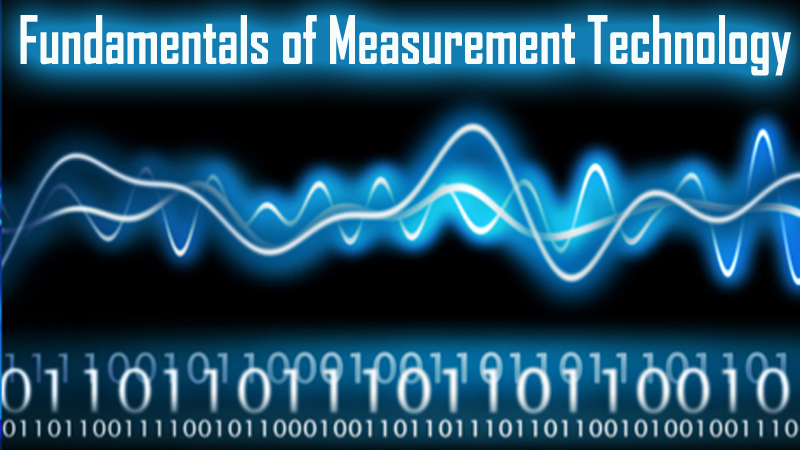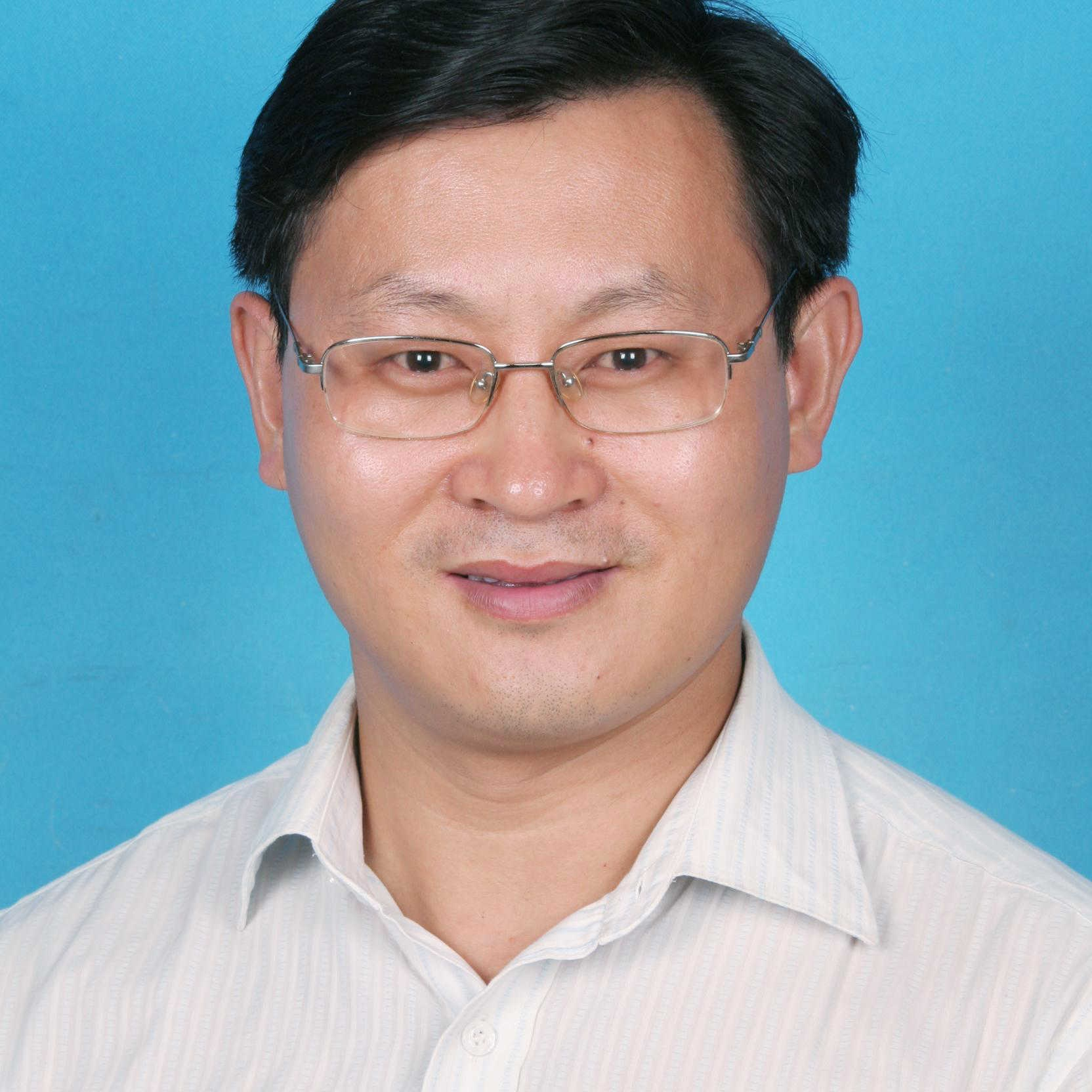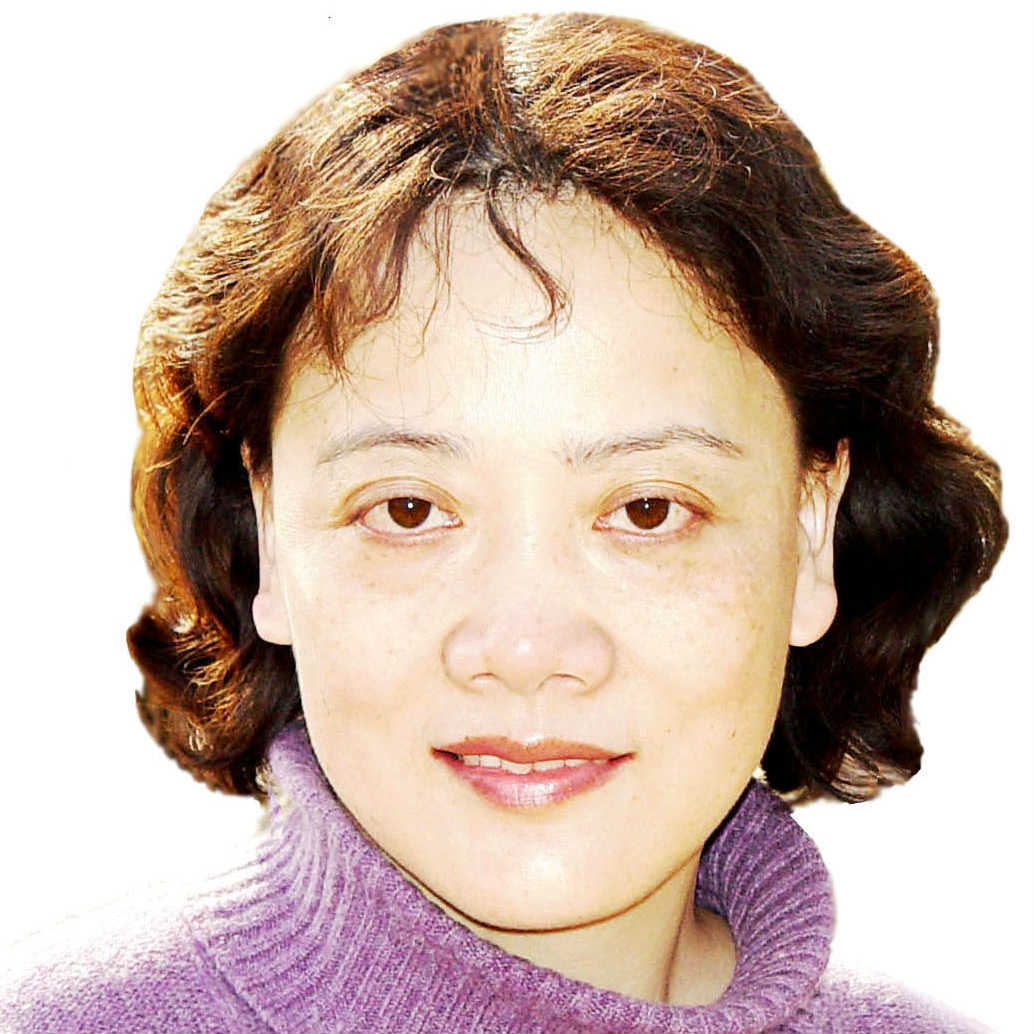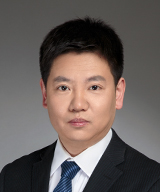# 测试与检测技术基础

## 测试与检测技术基础课程简介：前往报名学习## 测试与检测技术基础课程目录：

#### Week1 绪论 (Introduction)

--测试技术发展与研究内容 (The development of measurement technology)

--测量的本质与基本前提 (The precondition and foundation of measurement)

--标准及其单位 (Standards and Units)

--本章小结 (Chapter summary)

#### Week2 测试信号分析与处理（1）(Signal processing I)

--测试信号分析与处理基础知识 (Basic knowledge of signal processing)

--周期信号的频域描述 (Fourier series)

--非周期信号的频域描述 (Fourier transform)

#### Week3 测试信号分析与处理（2） (Signal processing II)

--傅里叶变换的性质 (The property of Fourier transform)

--功率信号的傅里叶变换 (Fourier transform of power signal)

--本章小结 (Chapter summary)

#### Week4 测试信号分析与处理（3）(Signal processing III)

--随机信号的描述 (Description of random signal)

--随机过程主要特征参数 (Characteristic parameters of stochastic process)

--相关分析 (Correlation analysis)

--功率谱分析与巴塞伐尔定理 (Power spectral analysis and Parseval’s theorem)

#### Week5 测试信号分析与处理（4） (Signal processing IV)

--数字信号处理概述 (Outline of digital signal processing)

--离散傅里叶变换 (Discrete Fourier transform)

--离散傅里叶变换的性质 (The property of discrete Fourier transform)

--采样定理 (Sampling theorem)

--泄漏与加窗 (Spectral leakage and windowing)

--栅栏效应 (Picket fence effect)

--快速傅里叶变换 (Fast Fourier transform)

#### Week6 测试系统特性分析（1） (Analysis of measurement system I)

--测试系统概述 (Outline of the measurement system)

--测量误差 (Measurement error)

--测试系统的静态特性 (Static characteristics)

--测试系统的动态特性 (Dynamic characteristics)

#### Week7 测试系统特性分析（2） (Analysis of measurement system II)

--伯德图与奈奎斯特图 (Bode plot and Nyquist plot)

--一阶惯性系统 (First-order system)

--二阶惯性系统 (second-order system)

--测试系统对典型激励的响应函数 (The response function of typical signal stimulus)

--测试系统实现精确测量的条件 (The preconditions of accurate measurement)

--测试系统的负载效应 (Loading effect)

#### Week8 被测量的获取（1） (Sensors I)

--被测量获取的基本概念 (Outline of sensors)

--传感器的分类 (The category of sensors)

--电阻式传感器 (Resistive sensors)

#### Week9 被测量的获取（2） (Sensors II)

--电感式传感器 (Inductive sensors)

--电容式传感器 (Capacitive Sensors)

--压电传感器 (Piezoelectric sensors)

--磁电式传感器 (Magnetic sensors)

#### Week10 被测量的获取（3） (Sensors III)

--霍尔传感器 (Hall sensors)

--图像传感器 (CCD image sensor)

--光纤传感器 (Fiber optic sensor)

--传感器选用原则 (Selection principles of sensors)

#### Week11 测试信号的转换与调理（1） (Signal conditioning I)

--测试信号转换绪论 (The introduction of signal conditioning)

--电桥 (Bridge circuit)

--调制与解调 (Modulation and demodulation)

#### Week12 测试信号的转换与调理（2） (signal conversion II)

--滤波器概述 (Outline of filter)

--滤波器的一般特性 (The characteristics of filter)

--滤波器的类型介绍 (The category of filter)

--滤波器的综合运用与MATLAB实现 (The application of filter and MATLAB realization)

--虚拟仪器技术概述

## 测试与检测技术基础授课教师：

### 王雪-教授-清华大学-精密仪器系### 李玉和-副教授-清华大学-精密仪器系### 罗秀芝-高级实验师-清华大学-精密仪器系### 杨兴-副研究员-清华大学-精密仪器系© 柠檬大学 2020